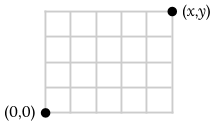# PS08: More Induction

Due Mon 4/20 @ 1:30pm.

The first set of problems below all relate to the Sierpinski Triangle. Here are some observations that might be helpful:

• The five figures shown in the book are the Sierpinski Triangles (all with side length 1) at levels $\ell = 0$, $\ell = 1$, $\ell = 2$, $\ell = 3$, and $\ell = 4$, respectively.
• The perimeter of a Sierpinski Triangle is the total length of all the black lines (that is, all the lines that separate a filled-in interior region from an empty white region).
• There are several ways to make a Sierpinski Triangle at level $\ell + 1$ from one at level $\ell$. The book tells you one (which I'll reiterate below), and there's another one that may be useful to you.

Before you work on these problems, study the figures in the book and make sure you understand how each of these different approaches works to generate the figures you see. (I'm using capitalization to distinguish “Sierpinski Triangles” (which may be riddled with triangular holes) and regular, lower-case “triangles” (which are solid figures).

• The book's way: make three copies of the level-$\ell$ Triangle, shrink them all down by a factor of 2 in each dimension, and then arrange them in a new triangle shape, with the points touching.
• Another way: for each little solid-grey triangle in the level-$\ell$ Triangle, find the midpoint of each of its sides, connect these three midpoints to form a new, even smaller triangle, and cut that out of the figure you had. (One fact to notice: the solid triangles that make up a level-$\ell$ Sierpinski Triangle are all the same size. The operation I described, performed on all such triangles, preserves the same-size property at level $\ell + 1$ too.)

Do these problems from the book, listed on pages 516. Note that each proof needs only a weak hypothesis!

1. 5.15 — Hint: define a value $\alpha$ that's equal to the area of the level-0 Triangle, and use it in all your computations. It makes your claim much easier to state, and the math in your proof much easier to follow.
2. 5.16

Do these problems from the book, listed on pages 527 and 528. These ones will be easier if you use a strong hypothesis.

1. 5.33
2. 5.34
3. 5.51

Also do these problems, listed on page 539.

1. 5.59 (Note that this problem is only talking about non-negative even numbers.)
2. 5.65 (Note that a “bitstring” is a string, or sequence, of bits. So both 100110 and 10011001 are bitstrings, and the latter is a palindromic bitstring.)
3. 5.66

Also do this problem.

1. My house is just a few blocks away from a cool bar/restaurant/bowling alley/theater in Minneapolis. The part of Minneapolis where I live is laid out like a grid. For the sake of argument, let's imagine my house as the origin, and the bar $x$ blocks east and $y$ blocks north, at the corner $(x,y)$. We're only going to consider situations where the bar is north or east of me; that is, $x, y \ge 0$.So let's say I want to walk to the bar, following the street grid. I only ever walk north or east, one block at a time. Even under this constraint, I still have lots of choices: I could walk north until I'm even with the bar (at $(0,y)$) and then walk east, or I could walk east to $(x,0)$ and then north, or I could kinda zig-zag up and over. In all, there are

$$\frac{(x+y)!}{x!y!}$$

different choices of unique paths from my house to the bar. (We'll learn how to come up with this formula later in the course; for now, you'll have to take my word for it.) Try this formula out on a few small examples and convince yourself that it's correct. (Keep in mind that $0! = 1$.)

Then, for your solution to this problem, prove that the above formula for the number of paths is correct for all $x,y \ge 0$.

Here are a few hints:

• Think about a recursive definition of a path from my house to the point $(x,y)$. Pay careful attention to the cases where one coordinate is 0 but not the other!

• In a lot of problems that involve recursive or inductive reasoning, you have a somewhat free choice of base cases. Think about it explicitly: what should the base case (or cases) be for this problem?

• It might be helpful to think of this problem in terms of a function $H(x,y)$ that evaluates to the number of distinct paths from my house to a bar at $(x,y)$. How could you compute the value of $H(x,y)$ just by doing some very simple math on the values of $H$ evaluated for a small number of other positions?

• Remember that a factorial is just a product of positive integers up to some stopping point. You can always “pick off the top” from such a product:

\begin{align} n! &= n \cdot (n-1) \cdot (n-2) \cdot \ldots \cdot 2 \cdot 1 \\ &= n \cdot \left[ (n-1) \cdot (n-2) \cdot \ldots \cdot 2 \cdot 1 \right] \\ &= n \cdot (n-1)! \end{align}

For the same reason, $(n+1)! = (n+1) \cdot n!$.

You can also always “stack one more on top”; this is just reading the above derivation from the bottom up:

\begin{align} n \cdot (n-1)! &= n \cdot \left[ (n-1) \cdot (n-2) \cdot \ldots \cdot 2 \cdot 1 \right] \\ &= n \cdot (n-1) \cdot (n-2) \cdot \ldots \cdot 2 \cdot 1 \\ &= n! \end{align}

And, of course, $(n+1) \cdot n! = (n+1)!$.